# 梯度下降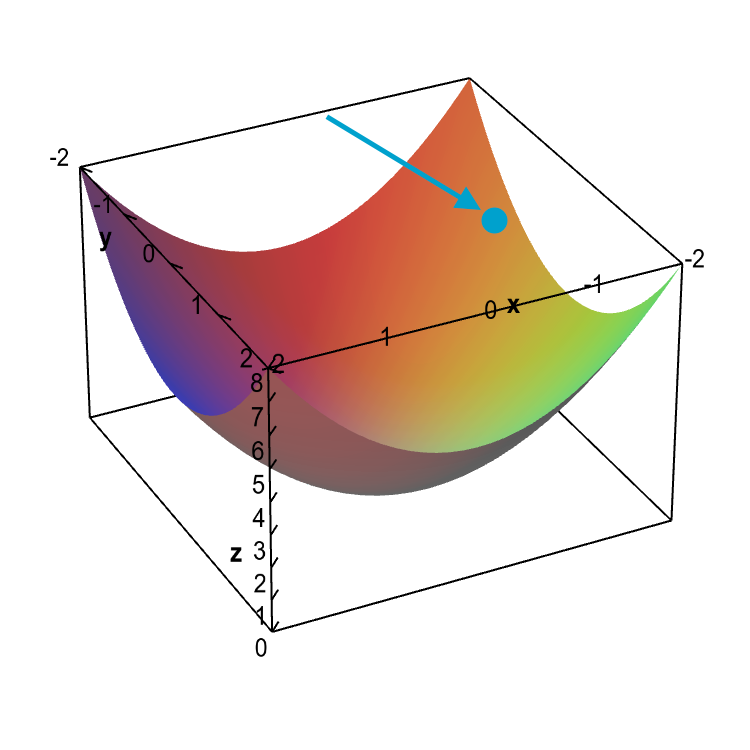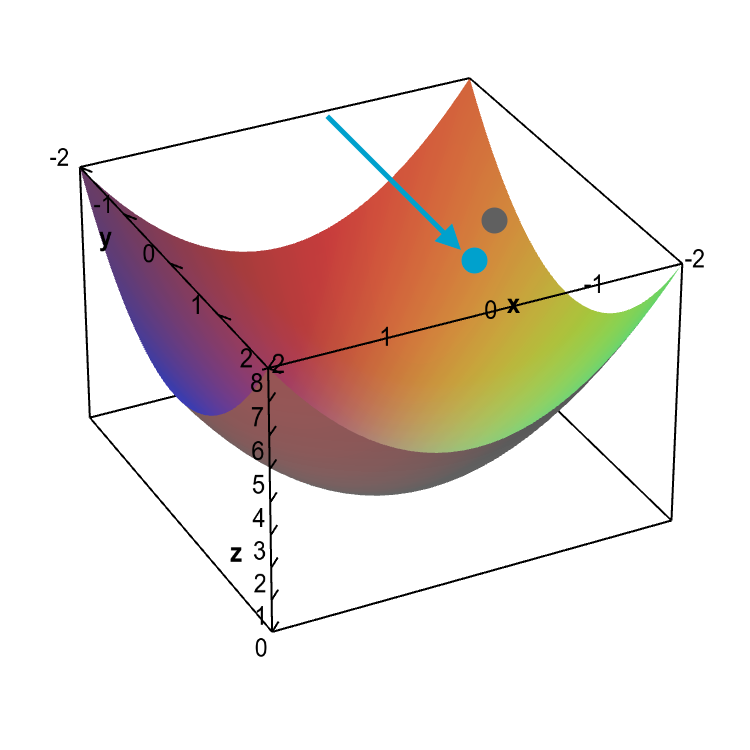# 在MiniFlow中集成梯度下降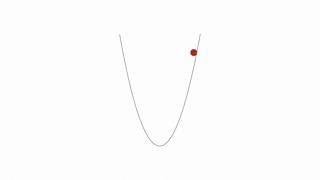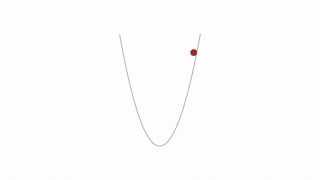``````x = x - learning_rate * gradient_of_x
#x表示神经网络中的某个参数，例如权重weight或者偏置bias
``````

# 梯度下降的代码实现

``````def gradient_descent_update(x, gradx, learning_rate):
"""
"""
# Return the new value for x
return x

``````

``````"""
Given the starting point of any `x` gradient descent
should be able to find the minimum value of x for the
cost function `f` defined below.
"""
import random

def f(x):
"""

It's easy to see the minimum value of the function
is 5 when is x=0.
"""
return x**2 + 5

def df(x):
"""
Derivative of `f` with respect to `x`.
"""
return 2*x

# Random number better 0 and 10,000. Feel free to set x whatever you like.
x = random.randint(0, 10000)
# TODO: Set the learning rate
learning_rate = 0.1
epochs = 100

for i in range(epochs+1):
cost = f(x)
print("EPOCH {}: Cost = {:.3f}, x = {:.3f}".format(i, cost, gradx))

``````

learning rate=0.1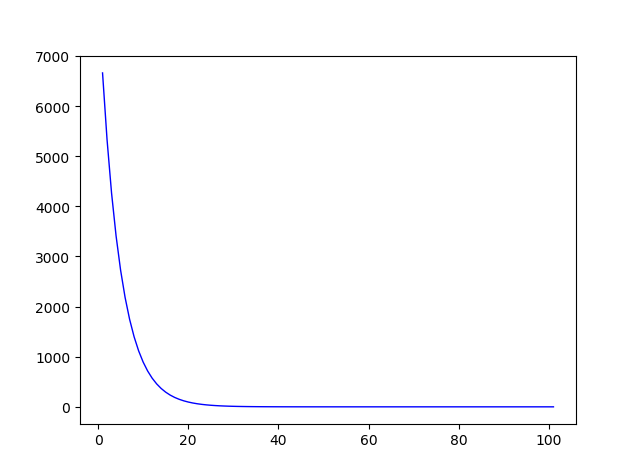learning rate=1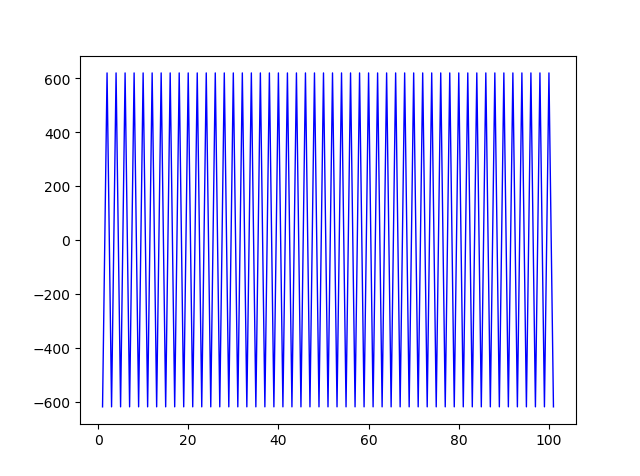Github链接